# Sample size & statistical power of a mean comparison test tutorial

2017-10-20

This tutorial will help you compute sample size and statistical power for a mean comparison test in Excel using the XLSTAT statistical software.

XLSTAT-Parametric tests includes several tests to compare means, namely the t and z tests. XLSTAT allows estimating the power of these tests and calculates the number of observations required to obtain sufficient power.

When testing a hypothesis using a statistical test, there are several decisions to take:

• The null hypothesis H0 and the alternative hypothesis Ha.
• The statistical test to use.
• The type I error also known as alpha. It occurs when one rejects the null hypothesis when it is true. It is set a priori for each test and is 5 %.

The type II error or beta is less studied but is of great importance. In fact, it represents the probability that one does not reject the null hypothesis when it is false. We cannot fix it up front, but based on other parameters of the model we can try to minimize it. The power of a test is calculated as 1-beta and represents the probability that we reject the null hypothesis when it is false.

We therefore wish to maximize the power of the test. XLSTAT calculates the power (and beta) when other parameters are known. For a given power, it also allows to calculate the sample size that is necessary to reach that power. The statistical power calculations are usually done before the experiment is conducted. The main application of power calculations is to estimate the number of observations necessary to properly conduct an experiment.

We place ourselves in a comparison of two independent samples. We want to know the number of observations required to obtain a power of 0.9 based on the t test based on the null hypothesis H0: Mean1 – Mean2 = 0. Since we do not yet know the parameters of our samples, we will use the concept of effect size. Cohen (1988) introduced this concept which provides an order of magnitude for the effect size, that is to say, the relative difference between the means. So we will test three effect sizes: 0.2 for a small effect, 0.5 for a moderate effect and 0.8 for a strong effect. As the effect size is based on the difference between the means, it is expected that for a greater effect, the sample size required will be smaller.

## Setting up of the calculation of the statistical power of a mean comparison test

After opening XLSTAT, click the Power icon and choose compare means.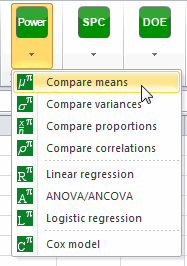Once the button is clicked, the dialog box appears. You must then choose the objective Find the sample size, then select the test t test for two independent samples, we take as the alternative hypothesis Mean 1 <> Mean 2. The alpha is 0.05. The desired power is 0.9. We suppose our samples are of equal size so the N1/N2 ratio is equal to 1. Rather than detailed input parameters, we select the effect size option and enter the value 0.2 for a weak effect.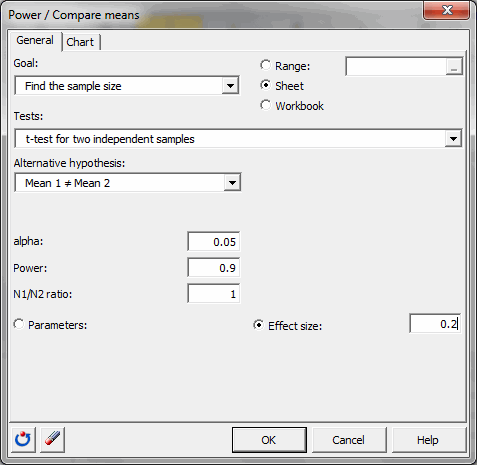In the Charts tab, the option simulation plot is activated and the “size of sample 1” will be displayed on the vertical axis and the “power” on the horizontal axis. Power varies between 0.8 and 0.95 by increments of 0.01.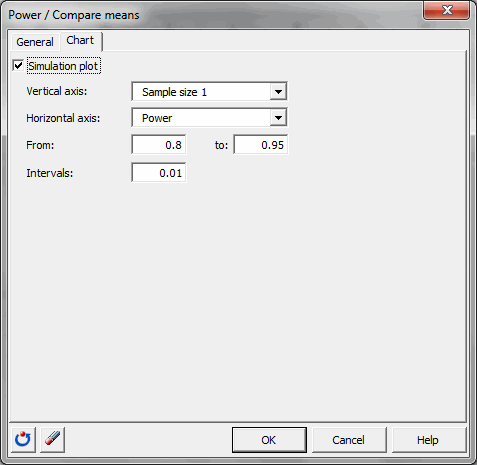Once you have clicked the OK button, the calculations are made, and then the results are displayed.

## Results of the calculation of the statistical power of a mean comparison test

The first table shows the calculation results and an interpretation of the results.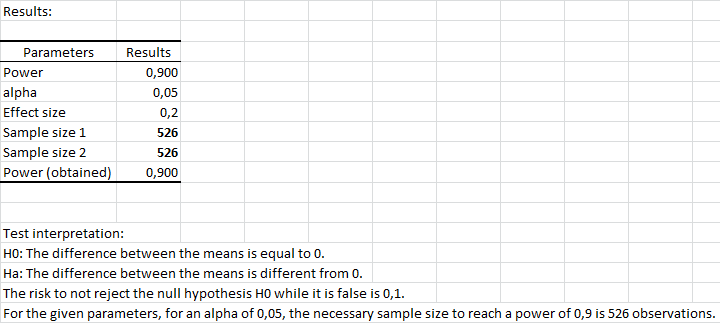We see it takes 526 observations per sample to obtain an output as close as possible to 0.9.

The following table summarizes the calculations obtained for each value of power between 0.8 and 0.95.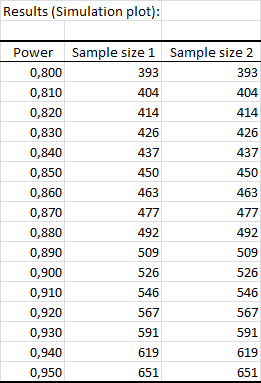The simulation plot shows the evolution of the sample size depending on the power. We see that for a power of 0.8, just slightly more than 393 observations per sample and as a power of 0.95 we get to 651 observations.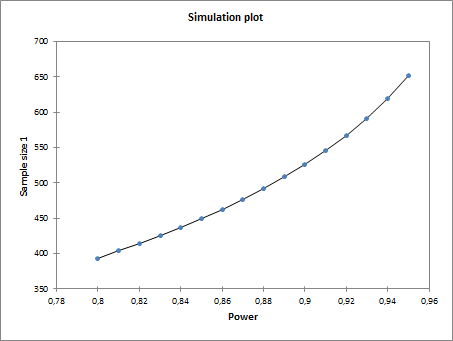For effect sizes of 0.5 and 0.8, we obtain the following results: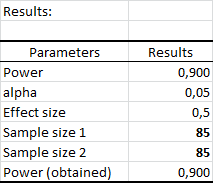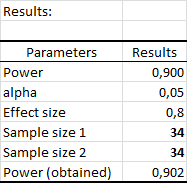The sample size will therefore fall as the difference between the means increases; we see that for a large difference, 34 observations per sample are sufficient.

XLSTAT is a powerful tool both to investigate the sample size required for an analysis and to calculate the power of a test. Obviously, if the user has more information about the samples or populations, he may give details of the input parameters, rather than using the effect size.

#### Contact our technical support team: support@xlstat.com

https://cdn.desk.com/
false
desk